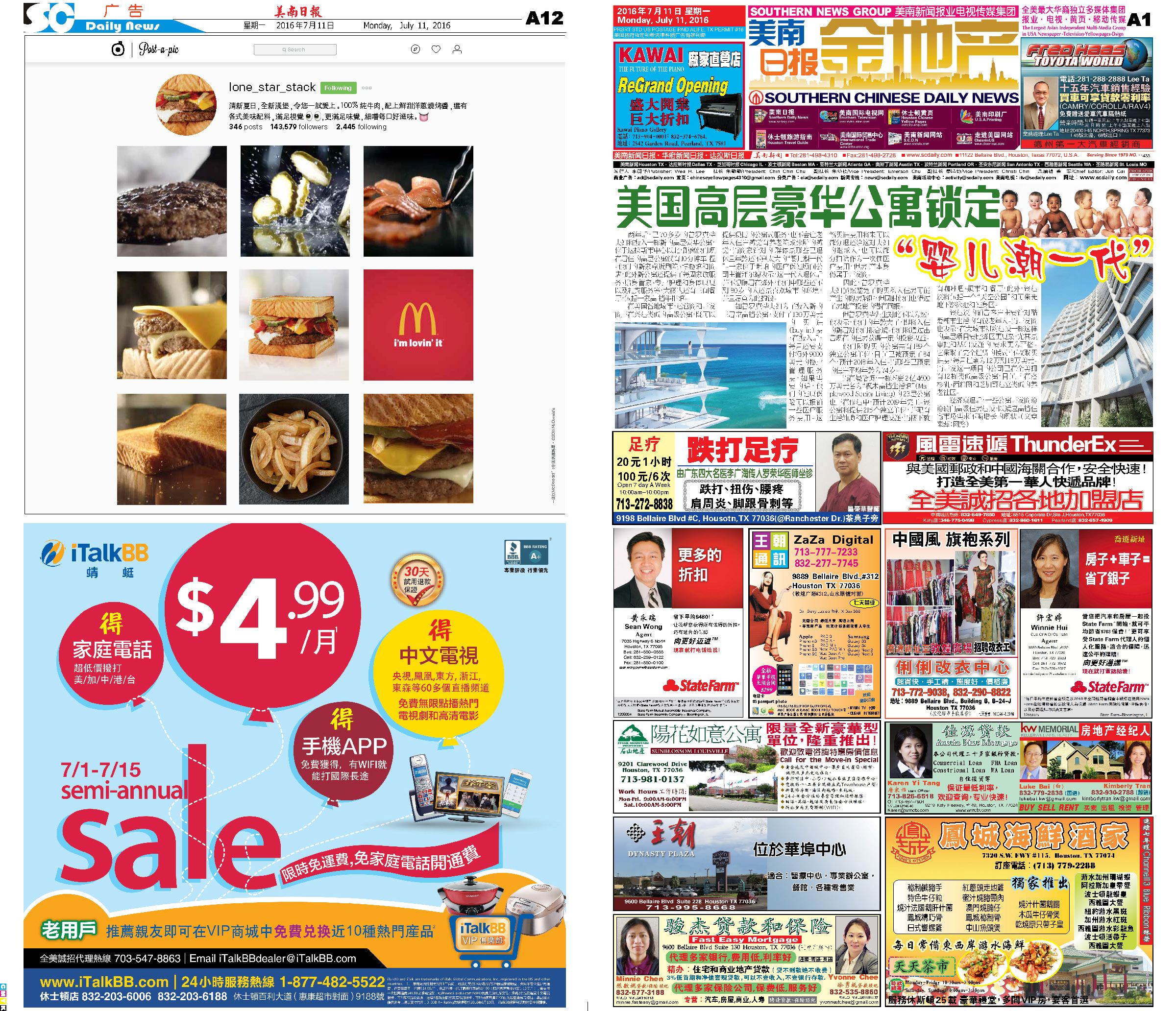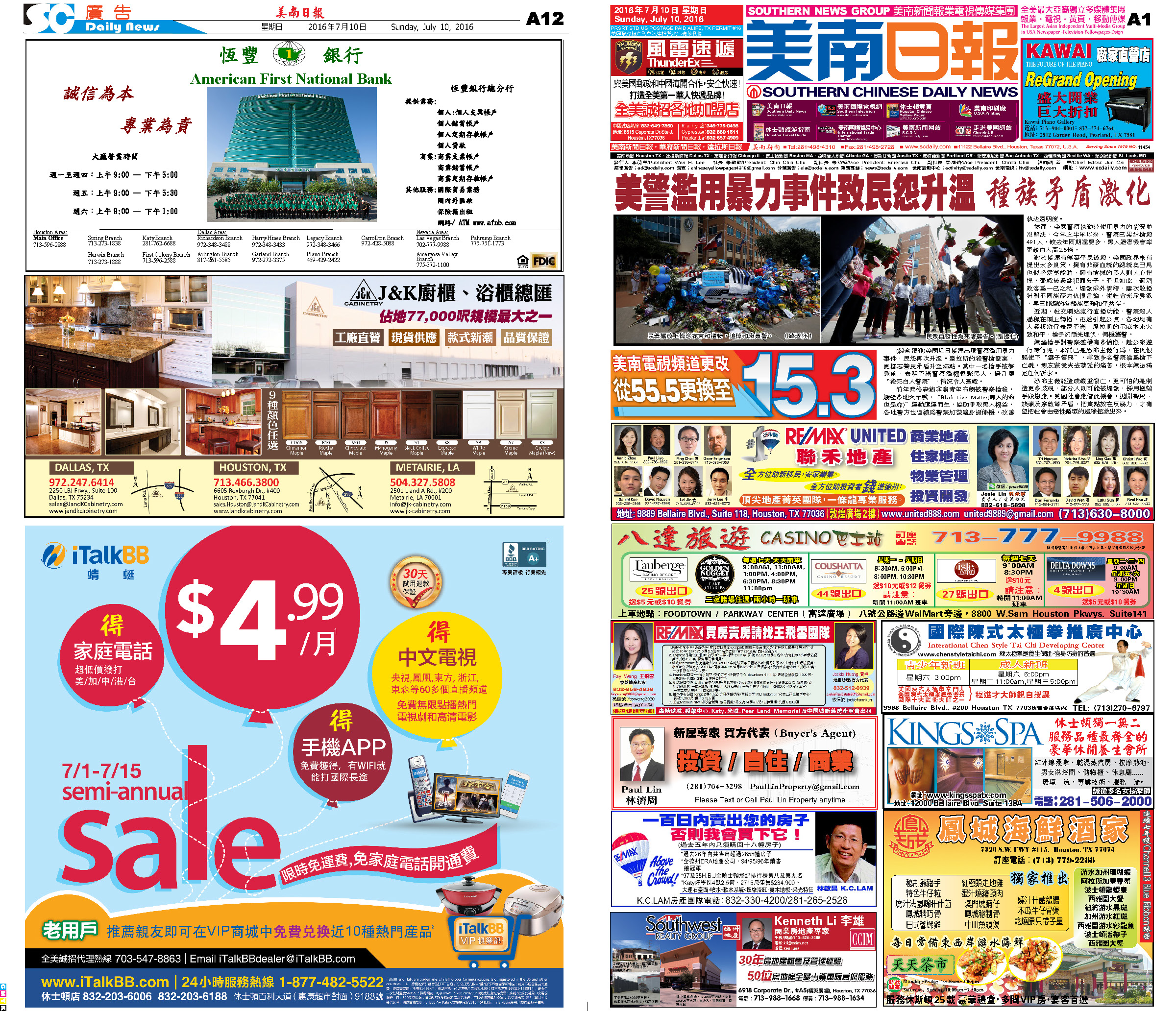160718 Epaper

 A Section B SectionC Section D Section160717 Epaper

 A Section B SectionC Section D Section160716 Epaper

 A Section B SectionC Section D Section160715 Epaper

 A Section B SectionC Section D Section160714 Epaper

 A Section B SectionC Section D Section160713 Epaper

 A Section B SectionC Section D Section160712 Epaper

 A Section B SectionC Section D Section160711 Epaper

 A Section B SectionC Section D Section160709 Epaper

 A Section B SectionC Section D Section160710 Epaper

 A Section B SectionC Section D Section160708 Epaper

 A Section B SectionC Section D Section